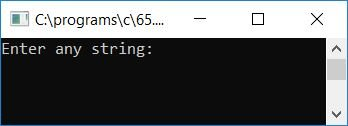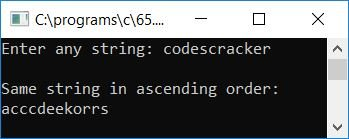# C program to sort strings

Here we will learn about how to sort any given string (by the user at run-time) in ascending and descending order, both one by one. Let's first start with how to sort strings in ascending order.

## Sort strings in ascending order using a C program

Here we have created a program that will receive any string from the user using the "gets()" function and then sort the string in ascending order. For example, if the given string is "codescracker," the string after sorting in ascending order will be "acccdeekorrs."

```#include<stdio.h>
#include<conio.h>
#include<string.h>
int main()
{
char str, chTemp;
int i, j, len;
printf("Enter any string: ");
gets(str);
len = strlen(str);
for(i=0; i<len; i++)
{
for(j=0; j<(len-1); j++)
{
if(str[j]>str[j+1])
{
chTemp = str[j];
str[j] = str[j+1];
str[j+1] = chTemp;
}
}
}
printf("\nSame string in ascending order:\n%s", str);
getch();
return 0;
}```

As the program was written under the "Code::Blocks" IDE, therefore, after a successful build and run, here is a sample run of the above program:Enter any string, such as "codescracker," and press the ENTER key to see the same string with its characters sorted ascendingly, as shown in the screenshot below:Here is a list of some of the main steps used in the above program:

• Receive any string using the "gets()" function.
• Get the length of a string using the strlen() function of the "string.h" library and initialize it to any variable, say "len."
• Now create a "for" loop that runs from 0 to one less than the string length.
• Inside the "for" loop, create another "for" loop with another loop variable, say "j," that runs from 0 to two less than the string length to compare each and every character of the string.
• When comparing, if any character in the string is greater than the next character, reverse the characters with each other and repeat until each character is arranged in ascending order.
• Therefore, we have run the loop two times. One time is up to one less than the string length, and the second time is up to two less than the string length.
• In this way, all the characters inside the string get arranged. We only have to print the string value, say "str."

## Sort the string in decreasing order using a C program

Let's create another program in C that will also receive any string from the user and sort the given string in descending order as shown in the program given below. For example, if the given string is "codescracker," then the string after sorting in descending order will be "srrokeedccca."

```#include<stdio.h>
#include<conio.h>
#include<string.h>
int main()
{
char str, chTemp;
int i, j, len;
printf("Enter any string: ");
gets(str);
len = strlen(str);
for(i=0; i<len; i++)
{
for(j=0; j<(len-1); j++)
{
if(str[j]<str[j+1])
{
chTemp = str[j];
str[j] = str[j+1];
str[j+1] = chTemp;
}
}
}
printf("\nSame string in descending order:\n%s", str);
getch();
return 0;
}```

Here is the final snapshot of its sample run:#### Other Programs on Sorting String

C Quiz

« Previous Program Next Program »